1. Worksheets>
2. Math>
3. Geometry>
4. Midpoint Formula

# Midpoint Formula Worksheets

Midpoint formula worksheets have a wide range of skills to find the midpoint of a line segment using number lines, grids and midpoint formula method. Also determine the missing coordinates, midpoint of the sides or diagonals of the given geometrical shapes, missing endpoints and more.

Midpoints on a number line

In these midpoint worksheets a number line is marked with numerous points. Find the midpoint of each indicated line segment.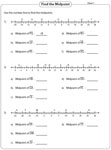Finding the midpoint: Grid

A line segment is represented on each grid. Identify the end points and find the midpoint of each line segment.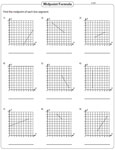Level 1:

Level 2:

Midpoint formula: Standard

Use the standard midpoint formula method to find the midpoint of a line segment with the given endpoints. Moderate level has endpoints in integers, fractions and decimals.Easy:

Moderate:

Midpoint and the shapes

Find the center of a circle, median of a triangle, point of intersection of diagonals of a square, rectangle, parallelogram and more.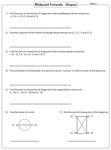Value of unknowns

The endpoints and the midpoint of a line segment are given with an unknown value. Find the value of the unknown.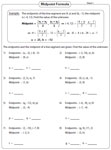Finding the end point: Standard

In these worksheets the one end of a line segment and the midpoint are given. Find the other end of the line segment.Finding the end point: Challenging

Find the described point with the two given reference points.

Eg. Find the point that is one-fourth of the way from (4, 2). The other end point is (8, 6).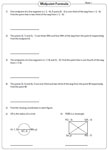The entire collection of midpoint formula worksheets can be downloaded in a jiffy!

Related Worksheets